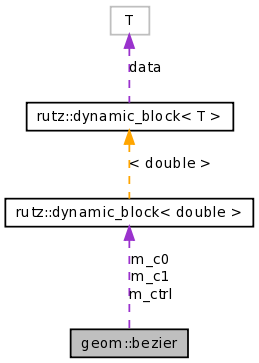# geom::bezier Class Reference

geom::bezier is a general class for evaluating Bezier curves More...

`#include <geom/bezier.h>`

Collaboration diagram for geom::bezier:[legend]

List of all members.

## Public Member Functions

bezier (const rutz::dynamic_block< double > &RR, int extrema_res=-1)
void set_control_points (const rutz::dynamic_block< double > &RR, int extrema_res=-1)
Reset the control points,.
double eval (double u)
Evaluate the Bezier curve for u in [0, 1].
double eval_deriv (double u)
Evaluate the derivative of the Bezier curve for u in [0, 1].
double arg_min ()
Get the arg-min value of the Bezier curve in the range [0, 1].
double arg_max ()
Get the arg-max value of the Bezier curve in the range [0, 1].
double eval_min ()
Get the min value of the Bezier curve in the range [0, 1].
double eval_max ()
Get the max value of the Bezier curve in the range [0, 1].

## Detailed Description

geom::bezier is a general class for evaluating Bezier curves

Also for evaluation Bezier derivatives, and their minimum and maximum values.

Definition at line 51 of file bezier.h.

## Constructor & Destructor Documentation

 geom::bezier::bezier ( const rutz::dynamic_block< double > & RR, int extrema_res = `-1` )

Construct from a vector of control points. The argument extrema_res is used to specify the number of subdivisions that will be examined when searching for the extrema. If extrema_res is < 0 (the default value), a default number of subdivisions will be used.

Definition at line 125 of file bezier.h.

References set_control_points().

The documentation for this class was generated from the following file:

The software described here is Copyright (c) 1998-2005, Rob Peters.
This page was generated Wed Dec 3 06:56:14 2008 by Doxygen version 1.5.5.